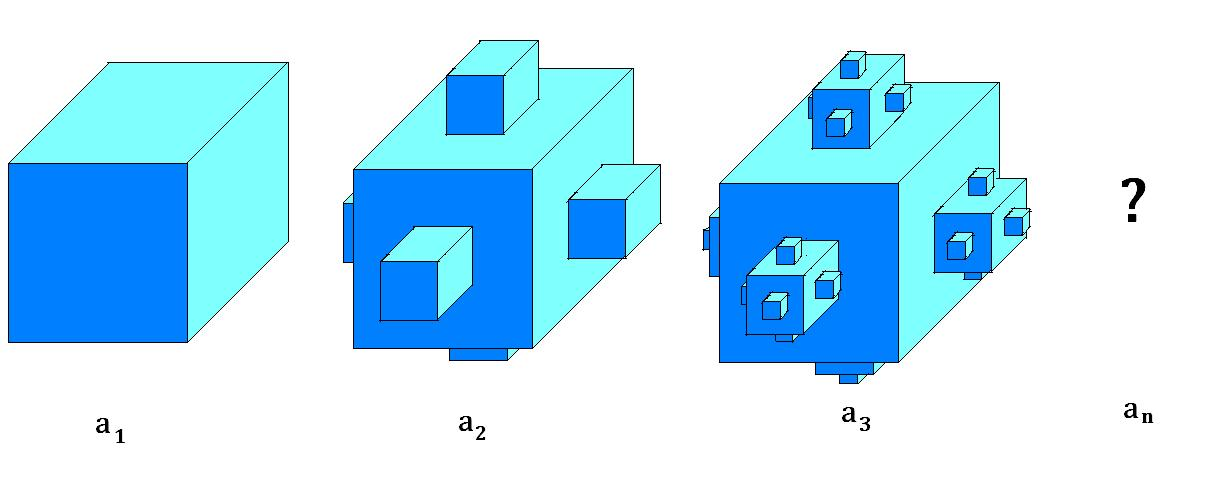Fractal Cubes

Probability Level 4The image above shows a construction of fractals by joining smaller and smaller cubes to each face of one single cube.

• We start with a cube of side length 3.
• In the second figure, the 6 new cubes are formed with a side length of $\dfrac{1}{3}$ of its previous cube.
• Then from the third figure and so on, the new cubes can only be formed on the 5 faces of the previous cubes with side length $\dfrac{1}{3}$ of its previous cube.

This recursion continues indefinitely.

If $a_{n}$ is the total surface area of the $n^\text{th}$ figure (from the left), what is the value of $\displaystyle \lim_{n \to \infty} a_{n}$?

×